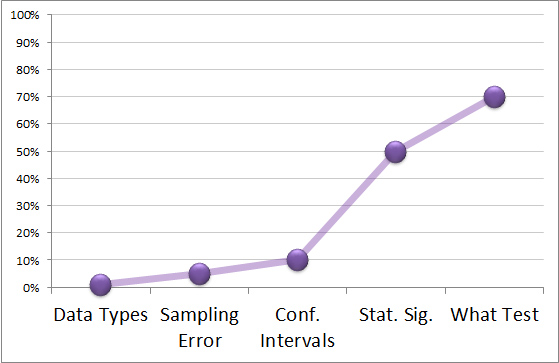Statistics can be daunting, especially for UX professionals who aren’t particularly excited about the idea of using numbers to improve designs.

But like any skill that can be learned, it takes some time to understand statistical concepts and put them into practice.

Most participants at our UX Boot Camp go from little knowledge of statistics to running statistical comparisons in just three days.

Here’s the path we take participants on.

## 1. Understand the Different Data Types

Not all data is created equal. You need to know what type of data you have to determine the best statistical test and method for finding the sample size.

While there are a number of ways to organize data into types, first segment the data into two groups: qualitative and quantitative. Qualitative data limits the type of statistical analysis you can perform, but at the very least you can still count, and summarize the counts of observations, names, and other non-numeric attributes.

Quantitative data can be subdivided into discrete and continuous groups. Discrete data is countable (e.g. number of errors) and is often binary (having only two options) such as purchased/didn’t purchase or complete/didn’t complete. But you can’t further divide discrete data into smaller units—you can’t have half a completion rate for example. Continuous data can be subdivided into smaller meaningful units. For example, task time is continuous and you can break down time from say a minute to 30 seconds, 1 second, etc.

Continuous data provides more fidelity and therefore requires a smaller sample size to detect differences. Where possible, look to collect more continuous measures; you can always decompose a continuous measure down to a discrete or binary measure.

## 2. Grasp Sampling Error

Rarely can you measure the entire population of users for a product or website. Instead you have to rely on a sample of users. For example, instead of surveying all 100,000 customers to see how likely they are to renew their service contract, we can use a sample of 100. The percentage of customers in the sample who state they will repurchase will fluctuate depending on the sample.

We might get a lot more interested customers than we would if we measured all 100,000 customers just from random chance. Interestingly enough, the mean or proportion we collect from samples, even rather small samples, doesn’t fluctuate as much as you might think. In fact, there’s a pattern to how much sample means fluctuate that allow us to better predict the unknown population mean. This is embodied in the most important concept in statistics, called the central limit theorem. It’s why techniques like confidence intervals and statistical tests work—they take into account sampling error.

## 3. Compute Confidence Intervals

To understand how precise an estimate is from a sample, you use a confidence interval. Confidence intervals take into account the sampling error and tell you the mostly likely range for the unknown population average.

You’ve almost certainly heard of the margin of error, usually in regard to an electorate’s attitude toward an issue. If 50% approve of the president’s job performance with a margin of error of 3%, the confidence interval is 47% to 53%. This range is the best estimate (usually from around 1,000 people) for how every eligible voter (millions of people) would also assess the performance.

With confidence intervals, you need to consider three factors:

• Sample size: The larger the sample size, the narrower the confidence interval.
• Confidence level: The more confident you need to be, the wider your interval will be.
• Variability: The more variable your sample is (as measured by the standard deviation), the wider the interval. For binary data like agree/disagree, the most variability happens at 50%.

## 4. Understand Statistical Significance

Statisticians continue to argue over the exact definition of statistical significance. In practice, statistically significant means a result is unlikely due to sampling error (chance variation). Most statistical tests present a probability value (called the p-value).

The p-value is the probability of obtaining a difference you see from a sample (or a larger one) if there really isn’t a difference for all users. For example, if we compare two designs with a sample of users and find the difference in completion rates is 20% and the p-value is .03, this tells us we’d only expect to see a difference of 20%, if there really was no difference (random noise), about 3 times in 100.

A conventional (and arbitrary) threshold for declaring statistical significance is when the p-value is less than 0.05. So in the example above, we’d say the difference in completion rates is statistically significant. Statistical significance doesn’t mean practical significance. Only by considering context can we determine whether a difference is practically significant.

## 5. Know What Statistical Test to Use

It takes years to know what statistical test to use. There are often multiple viable options. To choose the best statistical test, you need to know three key ingredients:

• Data type: Know your data type (step 1 from above). In most cases, you need to know whether your data is discrete-binary or continuous.
• Making a comparison: Whether you’re making a comparison (for example, comparing two designs) or estimating a population average.
• Within- or between-subjects: If you’re making a comparison, you need to know whether the exact same participants are in both samples (within-subjects) or if you’re using different participants (between-subjects).

While there are dozens of statistical tests, only a few address most scenarios. For example, comparing task times from two different sets of users on two designs, you’d use a 2-sample t-test (continuous, making a comparisons, between-subjects). If you want a confidence for a completion rate, you use the Adjusted-Wald confidence interval (discrete-binary and not making a comparison).